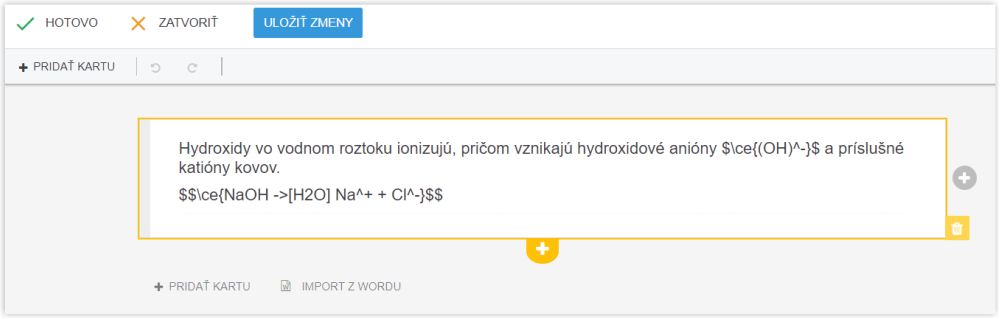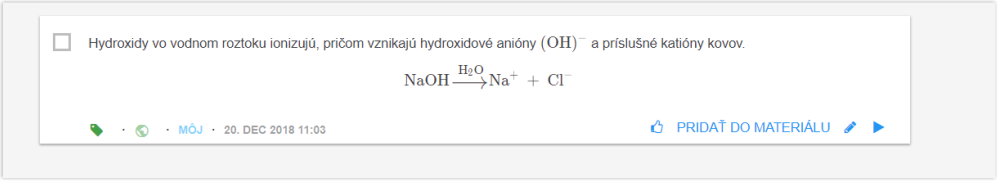EN# How to input chemical compounds

LaTeX is an instrument for writing mathematical expressions. The "mchem" part of this instrument is for writing chemical formulas.

Chemical formulas to be displayed in a line of text, have to be enclosed in dollar signs and curly brackets \ce, tj. \$ \ce{...} \$

Chemical formulas to be displayed in a separate line have to be enclosed in double dollar signs \$\$ \ce{....} \$\$

for example:Instructions for LaTeX users can be found on the internet: LaTeX math and equations , or LaTeX Wikibooks

Instructions for "mchem" users can be found here: LaTeX math and equations